# Passive and active ventricular elastances of the left ventricle

## Abstract

### Background

Description of the heart as a pump has been dominated by models based on elastance and compliance. Here, we are presenting a somewhat new concept of time-varying passive and active elastance. The mathematical basis of time-varying elastance of the ventricle is presented. We have defined elastance in terms of the relationship between ventricular pressure and volume, as: dP = EdV + VdE, where E includes passive (Ep) and active (Ea) elastance. By incorporating this concept in left ventricular (LV) models to simulate filling and systolic phases, we have obtained the time-varying expression for Ea and the LV-volume dependent expression for Ep.

### Methods and Results

Using the patient's catheterization-ventriculogram data, the values of passive and active elastance are computed. Ea is expressed as: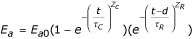; Epis represented as: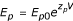. Ea is deemed to represent a measure of LV contractility. Hence, Peak dP/dt and ejection fraction (EF) are computed from the monitored data and used as the traditional measures of LV contractility. When our computed peak active elastance (Ea,max) is compared against these traditional indices by linear regression, a high degree of correlation is obtained. As regards Ep, it constitutes a volume-dependent stiffness property of the LV, and is deemed to represent resistance-to-filling.

### Conclusions

Passive and active ventricular elastance formulae can be evaluated from a single-beat P-V data by means of a simple-to-apply LV model. The active elastance (Ea) can be used to characterize the ventricle's contractile state, while passive elastance (Ep) can represent a measure of resistance-to-filling.

## Background

The heart may be conceived as a pump that receives blood from a low-pressure system and raises it to a high-pressure system. Although the mechanism responsible for generation of wall stress (and hence left ventricular (LV) pressure) is contraction of the myocardial fibers, an analytical formulation linking the myocardial and LV dynamics is still lacking. In the absence of this formulation, a popular way of linking LV pressure and volume dynamics is by means of LV compliance (or elastance) [1, 2]. Although this yields the cyclic values of elastance and compliance, this concept does not provide an intrinsic measure of elastance and compliance for the contractile state of the LV.

The concept of compliance or elastance was first employed for blood vessels , by relating incremental cross-section area (or volume) and transmural pressure. Warner appears to be have been the first to adopt a compliance description for a dynamic heart . In Warner's description, a mean value of compliance is adopted for diastolic phase and another mean value for systole, with abrupt transitions between the two states.

Defares  avoided the stepwise transition between diastolic and systolic compliance, by making elastance a continuously varying function of time. Later, the concept of a continuously varying compliance or elastance was adopted by a number of investigators with diverse variations [2, 6, 7]. Nevertheless, they all share the concept of a simple and extrinsic compliance term as an adequate description of ventricular mechanics during the cardiac cycle, based on monitored values of LV pressure and volume.

Classically, ventricular compliance is defined, at any point in time, as the change in ventricular volume concomitant with the change in ventricular pressure, such that

C = dV / dP, E = dP / dV     (1)

If C is assumed constant, this equation becomes a linear relation, whose integration gives

V = CP + V c     (2)

where Vc is an integration constant. If the compliance varies with time, then all terms in equation (2) may vary with time, as:

V (t) - V c (t) = C(t)P(t)     (3)

In this context, Suga  opted for the definition of ventricular elastance asto represent the elastance of the contracting LV, where Vd represents the unstressed LV volume corresponding to zero LV-pressure, obtained by drawing a tangent to P-V curves at end-ejection, as illustrated in Figure 1. This model gave rise to the development of the end-systolic pressure-volume relation (ESPVR) as a measure of contractility .

However, the determination of the maximal slope Emax and of the volume-axis intercept (Vd) of the tangent to the P-V curve at end-ejection (as a measure contractility of the cardiac muscle) is not only unreliable [11, 15], but also requires generation of multiple P-V loops under variations loading conditions [11, 15]. It is hence impractical to use clinically for a specific LV catheterization-ventriculography data. Above all, all of these variations in the concept of E obtained from LV pressure-volume data fail to explain the phenomena of LV suction and LV pressure drop during early filling as well as the generation of LV pressure increase during isovolumic contraction.

We have hence come up with a new concept of dual passive and active elastances operating throughout the cardiac cycle. The passive elastance (Ep) represents the LV pressure response to LV volume change (to LV volume increase during LV filling phase and to LV volume decrease during LV ejection phase). However, simultaneously, we also have active elastance (Ea) representing the contraction of the left ventricle due to its sarcomeric activation (and the development of force between the actin-myosin units) and relaxation (due to disengagement of the actin-myosin units).

LV Ea develops after the start of isovolumic contraction, becomes maximum some time during late ejection and thereafter decreases and becomes zero during diastolic filling. On the other hand LV Ep starts increasing after the initiation of LV filling as the LV volume increases. It reaches its maximum value at the end-of-filling phase, remains constant during isovolumic contraction, and thereafter decreases during ejection (as the LV volume decreases). While the generation of Ea helps us to explain the development of the LV pressure increase during isovolumic contraction, the decrease of Ea during diastole helps us to explain the decrease in LV pressure during early filling. The incorporation of both Ep and Ea helps us to explain the LV pressure changes during the filling and ejection phases.

## Methods

### Data acquisition

The subjects in this study were studied in a resting recumbent (baseline) state, after premedication with 100–500 mg of sodium pentobarbital by retrograde aortic catheterization. Left ventricular chamber pressure was measured by a pigtail catheter and Statham P23Eb pressure transducer; the pressure was recorded during ventriculography. Angiography was performed by injecting 30–36 ml of 75% sodium diatrizoate into the LV at 10 to 12 ml/s. It has been found by using biplane angiocardiograms that calculated orthogonal chamber diameters are nearly identical . These findings are used to justify the use of single-plane cine techniques, which allow for beat-to-beat analysis of the chamber dimensions.

For our study, monoplane cineangiocardiograms were recorded in a RAO 30° projection from a 9 in image intensifier using 35 mm film at 50 frames/s using INTEGRIS Allura 9 system at the Nation Heart Centre (NHC), Singapore. Automated LV analysis was carried out to calculate LV volume and myocardial wall thickness. The LV data, derived from the cineangiographic films and depicted in Figure 2 consists of measured volume and myocardial thickness of the chamber as well as the corresponding pressure. All measurements are corrected for geometric distortion due to the respective recordings systems.

In Figure 2, it is noted that during the early filling phase, LV pressure decreases even though LV volume increases. This phenomenon is defined as the 'LV suction effect', which will be explained later by using our concepts of active and passive elastances. This phenomenon is also depicted in Figure 3 and Table 1.

### Definition of passive elastance and active elastance of the LV

At the start of diastolic-filling phase, the LV incremental pressure dPLV is the response to (i) LV Ea continuing to decrease due to the sarcomere continuing to relax well into the filling phase, and (ii) to the rapid inflow of blood and the corresponding increase in LV volume, along with increase in LV Ep. The associated governing differential equation, relating LV pressure and volume, can be put down (by referring to the Appendix for its derivation) as :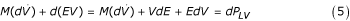where t represents the time variable (s) from the start of filling phase;

V represents the volume of LV (ml) during the filling phase;

P LV represents pressure of the LV, in mmHg (hereafter symbolized by P) (mmHg);

M represents the inertia term = [LV wall-density (ρ)/(LV surface-area/wall-thickness)] = ρh / 4πR2, for a spherical LV model (in mmHg/(ml/s2));

E represents LV elastance (mmHg/ml).

Likewise during ejection, the LV pressure variation (dPLV) is caused by both Ea variation as well as Ep decrease. The instantaneous time-varying ventricular elastance (E) is the sum of (i) volume-dependent passive elastance (Ep) and (ii) active elastance (Ea) due to the activation of the LV sarcomere. Hence,

E = E a + E p     (6)

We will now provide the expressions for Ep and Ea, and then their formulations. The passive (unactivated) myocardium exhibits properties of an elastic material, developing an increasing stress as strain increases, as occurs during ventricular filling. The passive stress-strain relation of myocardial muscle strip is nonlinear, and therefore cannot be described by Hooke's law. As an approximation, it follows an exponential relationship . Therefore, the relation between LV passive pressure and volume has also been assumed to be exponential. Since Ep (= dP/dV) is volume-dependent, we can express it as: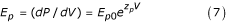where Ep 0is the passive elastance coefficient, z p is the passive elastance exponent, and V is the LV volume; its derivation is provided in a subsequent section.

On the other hand, we will represent E a as an intrinsic property of the LV (derived later), as:where (i) t is measured from the start of isovolumic contraction, (ii) the parameter Ea 0is the active elastance coefficient, (iii) the time-coefficient (τ C ) describes the rate of elastance rise during the contraction phase, while (τ R ) describes the rate of elastance fall during the relaxation phase; (iv) the exponents "Z C " and "Z R " are introduced to smoothen the curvatures of the E a curve during isovolumic contraction and relaxation phases; (v) the parameter d is a time constant whose (to be determined) value is during the ejection phase, and (vi) u(t-d) is the unit step function, u(t-d) = 0 for t<d. The rationale for equation (8), as provided in the next section, is based on Ea incorporating parameters reflecting the (i) generation of LV pressure during isovolumic contraction, (ii) decrease of LV pressure during isovolumic relaxation and early filling, and (iii) the LV pressure-volume relationship during filling and ejection phase.

### Our hypothesis

Based on equations (5–8), our hypothesis is that both Ea and Ep contribute to the relationship of LV pressure and volume. While Ep incorporates LV pressure change due to LV volume change, Ea incorporates the effect of LV myocardial activation in the generation of LV pressure during the isovolumic phases (when the LV volume remains constant). Since Ea is deemed to be the basis of LV pressure generation, its variation (as given by equation 8) corresponds to the LV pressure variation.

### Determination of Ea and Ep expressions

#### a) Active elastance (during isovolumic contraction and relaxation)

During isovolumic contraction (because dV = 0,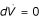and Ep is constant), the governing equation (5) becomes VdE = dP LV , which can be detailed as:

V i (E i - Ei-1) = V i [(E a,i + E p,i ) - (Ea,i-1+ Ep,i-1)] = V i (E a,i + E ped - Ea,i-1- E ped ) = dP LV,i     (9)

where i is a time instant during the isovolumic contraction and relaxation, Vi and PLV,i are the monitored LV volume and pressure at this instant, and Eped is the passive elastance at the end-diastolic phase.

Also, during isovolumic relaxation (because dV = 0,and Ep is constant), the governing equation (5) becomes VdE = dP LV , which can be represented as:

V i (E a,i + E p,i - Ea,i-1- Ep,i-1) = V i (E a,i + E pes - Ea,i-1- E pes ) = dP LV,i     (10)

where Epes is the passive elastance at the end-systolic phase

Now, applying equations (9 & 10) to the case shown in the Figure 2, we have (using the monitored LV pressure-volume data):

1. For isovolumic contraction

Ea,1= 0     (11-a)

Ea,2= (P2 - P1)/V2 + Ea,1= 0.029477 mmHg/ml     (11-b)

Ea,3= (P3 - P2)/V3 + Ea,2= 0.103771 mmHg/ml     (11-c)

Ea,4= (P4 - P3)/V4 + Ea,3= 0.253584 mmHg/ml     (11-d)

Ea,5= (P5 - P4)/V5 + Ea,4= 0.463599 mmHg/ml     (11-e)

2. For isovolumic relaxation

Ea,18= (P18 - P17)/V18 + Ea,17= Ea,17- 0.058954 mmHg/ml     (11-f)

Ea,19= (P19 - P18)/V19 + Ea,18= Ea,17- 0.177824 mmHg/ml     (11-g)

Ea,20= (P20 - P19)/V20 + Ea,19= Ea,17- 0.312656 mmHg/ml     (11-h)

Ea,21= (P21 - P20)/V21 + Ea,20= Ea,17- 0.463599 mmHg/ml     (11-i)

Now in the above expressions 11(f-i), Ea,17 at end-ejection is unknown. For different representative values of Ea,17, we can get different Ea curves. We need to determine the optimal value of Ea,17, such that Ea can be described by a smooth curve to fit both isovolumic contraction and ejection phases. In Figure 4, we have determined Ea,17 = 1.1 mmHg/ml, and the polynomial expression for Ea(t) to fit its above calculated values during isovolumic contraction and relaxation. However, in order to (i) more suitably represent Ea to correspond with its role during the cardiac phases (isovolumic, ejection and filling), and (ii) because of the sigmoidal shape of Ea curve and its variation resembling the LV pressure variation during systole (as seen in Figure 4), we express Ea (according to equation 8) as:So that its constituent parameters have physiological significance as indicated following equation (8).

Hence, to compute Ea(s) during isovolumic contraction, when u(t-d) = 0, we employ the expression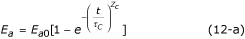and determine its parameters Ea0, τ C and ZC to fit the monitored pressure-volume data. Then, to compute Ea(s) during isovolumic relaxation, we employ the total expressionand determine its remaining parameters d, τ R and ZR to fit the measured pressure-volume data.

For the sample data of Figure 2, the variation of Ea is depicted in Figure 5, along with the values of its parameters. We now propose that Ea can be employed as an index of contractility.

#### b) Passive elastance determination during diastolic filling

During the diastolic filling phase, equation (5) becomesNow because Ep is constant at a particular volume Vi, equation (13) becomeswhere i is a time-instant during diastolic filling, Vi and PLV,i are the monitored LV volume and pressure at this time, and M = ρh / 4πR2. For the patient data (shown in Figure 2), we can get the mean value for M during diastolic filling, M = 8.03 × 10-6 mmHg/(ml/s2). Therefore, from equation (15), we can calculate the values of Ep at various instants during filling phase. We then plot Ep vs V, in Figure 6. By fitting equation (7) to these calculated values of Ep, we obtain the values of the parameters Ep 0and z p , as:

z p = 0.0395 ml-1, Ep 0= 4.375 × 10-3 mmHg / ml     (16)

and the Ep function (corresponding to its expression given by equation 7) as follows:

E p = 4.375 × 10-3 e0.0395V    (17)

We now propose to adopt Ep as a measure of LV resistance-to-filling.

Hence during ejection, both Ea and Ep are varying. During ejection and filling phases, Ep can be calculated at any time using equation (17). Likewise, Ea can be calculated during ejection and filling phases using equation (8), once its parameters have been determined by employing equation (12-b & 12-b) during isovolumic contraction and relaxation phases. Their values during the cardiac cycle are listed in Table 1.

## Clinical application results

The analyses, presented herewith, are now applied to clinically obtained data consisting of the subject's left ventricular (instant-to-instant) dimensions (obtained by cineangiocardiograph) and chamber pressure (obtained by cardiac catheterization). For each subject, passive and active elastances are determined from the left ventricular data. Table 2 provides the measured data and the model-derived parameters for three subjects (subject HEL, DDM, and ML). Subject HEL serves as a sample patient with myocardial infarct, subject DDM with double vessel disease (DVD) and hypertension, treated with PTCA; subject ML with idiopathic myocardial hypertrophy (IMH).

The variations of model-derived nonlinear passive and active elastances for the subject HEL are shown in Figure 7. For this particular subject (HEL), the maximum active elastance is 1.37 mmHg/ml. In Figure 8, we have plotted Ea vs incremental pressure (P-Ped) for this patient HEL. Note that the elastance is much higher at late-ejection than early ejection. This is because of a continuing sarcomere stress development and shortening. The active elastance reaches its maximum value at late-ejection (point 15), and thereafter decreases. However as shown in Figure 7, even after the end of relaxation phase (point 21) the active elastance continue to decrease into the filling phase. This decrease can explain the suction effect during the rapid filling sub-phase, even after LV filling has commenced.

Figures 9 and 10 provide representations of the nonlinear passive and active elastance for subject HEL (with hypertension), DDM (myocardial infarct), and ML (idiopathic myocardial hypertrophy). The Ep vs LV volume plots, in Figure 9, clearly reveal that Ep increases exponentially with increase LV volume; the parameters (EP 0and z p ) characterize this relationship. The passive elastance curve is steeper for a stiffer myocardium, with a corresponding bigger value of the exponential coefficient Z p (subject ML). The Ea vs incremental pressure (P-Ped) plots, in Figure 10, reveal the development and decrease of Ea during systole, which in turn governs the generation of LV pressure.

## Discussion

### Ea as a contractility index

Yet another way to study Ea variation is by means of the Ea vs normalized time (t/ts) plot, shown in Figure 11. These two figures 10 and 11 make us realize that Ea,max could be regarded as an index of LV contractility. Hence, we decided to plot Ea,max vs the traditional contractility indices of EF and (dP/dt)max. These plots are displayed in Figures 12 and 13. It is noted that Ea,max has a high degree of correlation with both EF and (dP/dt)max. It is interesting to compare our correlation-coefficient value (0.8972) with the value of 0.89 obtained by Mehmel et al , although this paper computes elastance as an extrinsic property = [P/(V-Vd)]es.

### Demonstrating LV suction phenomenon

The active elastance curve can explain some critical LV physiological phenomena, namely LV pressure generation during isovolumic contraction and LV suction during early filling. The rapid decrease in elastance during isovolumic relaxation extends into the filling phase, and can explain the decrease in LV pressure (in Figure 7) even after LV filling has commenced. Let us show how this happens, by rewriting equation (15) as follows:

Pi - Pi-1 = (Ep,i + Ea,i)(Vi - Vi-1) + Vi (Ea,i - Ea,i-1)     (18)

by neglecting the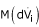term, based on the calculation of its value being of the order of 10-2 compared to (i) (E p,i + E a,i )(V i - Vi-1), which is of the order of 100 and (ii) V i (E a,i - Ea,i-1) which is the order of 101.

In equation (18), it is seen that Pi can be less than Pi-1 (or that Pi - Pi-1 < 0) only if (Ea,i - Ea,i-1) is negative, i.e., active elastance is decreasing. Now for subject H.E.L (Figure 1), the computed values of Ea and Ep at these 2 instants (based on equations 8 & 17) are:

Ep,22= 0.2768 mmHg/ml, Ea,22= 0.0393 mmHg/ml, Ea,21= 0.1140 mmHg/ml, V22 = 105 ml, V21 = 90.6 ml     (19)

Substituting these values into equation (18) gives (P22 - P21) = -3.22 mmHg, confirming decrease of pressure during early filling.

Hence, our novel concept of "decreasing Ea during the early phase of filling" enables us to explain the phenomenon of decreasing LV pressure during the early stage of filling. In other words, it is suggested that the sarcomere actin-myosin activity continues into the filling phase. The decreasing Ea during the filling phase seems to reflect decreasing sarcomeric activity during filling. Likewise, the increase in Ea during isovolumic contraction is responsible for increase in LV pressure at constant volume, as demonstrated by means of equation (11).

### Demonstrating variation of LV pressure during ejection in terms of Ea and Ep

Similarly, both the active and passive elastances can explain LV pressure variation during ejection, using equation (18). Let us show how this happens by just taking two instants (t10 & t9), as follows:

P10 - P9 = (Ep,10 + Ea,10)(V10 - V9) + V10(Ea,10 - Ea,9)     (20)

Substituting these computed values (listed in Table 1) into equation (20) gives P10 - P9 = 1.92 mmHg, which is approximately equal to the actual value of 2 mmHg.

Previous works have described elastance as a derived parameter from LV pressure-volume data, based on the definition of P / (V - V d ) [9, 10, 1214]. Also, Emax (given by ESPVR) as illustrated on Figure 1 varies with different arterial loading states and is therefore load dependent , and not an intrinsic index independent of LV loading states.

Our definitions of Ep and Ea enable us to explain the phenomena of (i) LV suction during early filing, (ii) LV pressure rise during isovolumic contraction (iii) LV pressure variation during the ejection phase, and (iv) LV pressure drop during the relaxation phase. Both the concepts of Ea and Ep are made possible by our redefining elastance and compliance as

dP = d(EV) = d(V/C) = VdE + EdV     (21)

Our concept of Ea enables us to explain (i) the generation of pressure during isovolumic contraction and decrease of pressure during isovolumic relaxation, when the volume is constant, and (ii) the decrease of pressure during the rapid filling phase.

Our concept of decreasing Ea along with increasing Ep during filling, which enables us to explain the LV suction effect during early filling, is indirectly supported by the work of Shoucri . He has indicated that the LV wall stress during the filling phase is made up of two components: (i) a passive increasing component due to LV pressure and (ii) an active decreasing component due to decreasing active fiber stress.

However, the determination of this active fiber stress in his paper is empirical and not based on a well-defined concept and expression for Ea, as provided by us.

Finally, both Ea and Ep are invoked to explain the variations of pressure during the ejection and filling phases. From the viewpoint of intrinsic indices of LV assessment, Ep can represent LV myocardial stiffness property and resistance to LV filling. On the other hand, Ea has been shown to correspond to LV contractility, by means of Figures 12 & 13. Therein, we have shown a high degree of correlation between Ea,max and (dP/dt)max as well as EF.

In future, we can also couple our LV model (of Ep and Ea) with an arterial lumped-parameter model (consisting of total peripheral resistance R, total arterial compliance C, aortic characteristic impedance Z0 and inertial L) in order to simulate and explain the mechanisms of chronic hypertensive states , in terms of alteration in E a itself as a measure of LV adaptation to chronic hypertension induced in the circulation system.

## Appendix: Derivation of Equation (5)

Dynamic equilibrium of the LV myocardial element gives (based on figure 14):where the myocardial element mass, m e = m s A e , m s (the myocardial surface-density or mass per unit surface area) = ρh, ρ is the myocardial density, and u is the radial displacement, dP el and dP LV are the incremental elastic-recoil and left ventricular pressures (as depicted in Figure A-1).

Now since,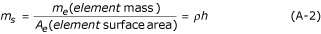By assuming r1 = r2 = Rwe can write:where ρ s is the surface density, M = ρ s h, and V is the LV volume. Now, referring to Figure A-1,and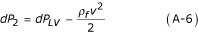where R e is the resistance to LV filling (through the open mitral-valve), ρ f is the blood density and v is the blood velocity at site 2.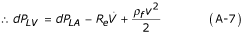Let us now define incremental elastic recoil pressure (in response to incremental LV pressure dP LV ) as:Hence, from equations (A-1, A-4, A-7 & A-8), we have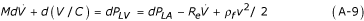Introducing the term elastance (= 1/C), we can put down## References

1. Noordergraaf A, Melbin J: Introducing the pump equation. In The heart and cardiovascular system-scientific foundations. Edited by: Haber E, Jennings RB, Katz AM, Morgan HE. New York: Raven Press; 1994:19–35.

2. Palladino JL, Mulier JP, Noordergraaf A: Defining ventricular elastance. Proc 20th Int Conf IEEE Eng Med Biol Soc, Hong Kong 1998, 383–386.

3. Noordergraaf A: Circulatory system dynamics. New York: Academic Press; 1978.

4. Warner HR: The use of an analog computer for analysis of control mechanisms in the circulation. Proc IRE 1959, 47: 1913–1916.

5. Defares JG, Osborn JJ, Hara HH: Theoretical synthesis of the cardiovascular system. Acta Physiolo Pharmacol Neerl 1963, 21: 189–265.

6. Suga H, Sagawa K: Instantaneous pressure volume relationships and their ratio in the excised, supported canine left ventricle. Cir Res 1974, 35: 117–126.

7. Kennish A, Yellin E, Frater RW: Dynamic stiffness profiles in the left ventricle. J Appl Physiol 1975, 39: 665–671.

8. Suga H: Time course of left ventricular pressure-volume relationships under various end-diastolic volume. Jap Heart J 1969, 10: 509–515.

9. Suga H, Sagawa K, Shoukas AA: Load independence of the instantaneous pressure-volume ratio of the canine left ventricle and effects of epinephrine and heart rate on the ratio. Circ Res 1973, 32: 314–322.

10. Suga H, Sagawa K: Instantaneous pressure volume relationships and their ratio in the excised, supported canine left ventricle. Circ Res 1974, 35: 117–126.

11. Sagawa K: The ventricular pressure-volume diagram revisited. Circ Res 1978, 43: 677–687.

12. Mehmel HC, Stochins B, Ruffmann K, Olshausen K, Schuler G, Kubler W: The linearity of the end-systolic pressure-volume relationship in man and its sensitivity for assessment of left ventricular function. Circulation 1981, 63(6):1216–1222.

13. Little WC, Cheng CP, Mumma M, et al.: Comparison of measures of left ventricular contractile performance derived from pressure-volume loops in conscious dogs. Circulation 1989, 95: 2423–2433.

14. Shishido T, Hayashi K, Shigemi K, Sato T, Sugimachi M, Sunagawa K: Single-beat estimation of end-systolic elastance using bilinearly approximated time-varying elastance curve. Circulation 2000, 102: 1983–1989.

15. Suga H: Paul Dudley white international lecture: cardiac performance as viewed through the pressure-volume window. Jpn Heart J 1994, 35: 263–280.

16. Sandler H, Dodge HT: The use of single plane angiocardiograms for the calculation of left ventricle volume in man. Am Heart J 1968, 75: 325–334. 10.1016/0002-8703(68)90089-6

17. Zhong L, Ghista DN, Ng EYK: Index for cardiac dysfunction of left ventricular filling phase. In Proceedings of the 5th International Conference on Simulations in Biomedicine: 2–4 April 2003. Volume 5. Ljubjana. WIT Press; 2003:209–218.

18. Mirsky I: Assessment of passive elastic stiffness of cardiac muscle: mathematical concepts, physiologic and clinical considerations, directions of future research. Prog Cardiovasc Dis 1976, XVIII(4):277–308. 10.1016/0033-0620(76)90023-2

19. Mirsky I, Pasipoularides A: Clinical assessment of diastolic function. Prog Cardiovasc Dis 1990, 32: 291–318. 10.1016/0033-0620(90)90018-W

20. Gilbert JC, Glantz SA: Determinants of left ventricular filling and of the diastolic pressure-volume relation. Circ Res 1989, 64(5):827–852.

21. Mulier JP: Ventricular pressure as a function of volume and flow. PhD thesis. University of Leuven; 1994.

22. Shoucri RM: Active and passive stresses in the myocardium. Am J Physiol Hear Circ Physiol 2000, 279: H2519-H2528.

23. Segers P, Stergiopulos N, Schreuder JJ, Westerhof BE, Westerhof N: Left ventricular wall stress normalization in chronic pressure-overloaded heart: a mathematical model study. Am J Physiol Heart Circ Physiol 2000, 279: H1120-H1127.

## Acknowledgements

We are grateful to Dr. Terrance S.J. Chua and Technician Mr. Ng Eng Hian from National Heart Centre, Singapore, for his technical assistance in cineangiographic recordings. This study was supported by grants from Strategic Development Scheme, Nanyang Technological University, Singapore.

## Author information

Authors

### Corresponding author

Correspondence to Dhanjoo N Ghista.

### Authors' contributions

Liang Zhong carried out the elastance studies, participated in data acquisition, performed statistical analysis and drafted the manuscript. Dhanjoo N. Ghista conceived of the study, and participated in its design and coordination and helped to draft the manuscript. Eddie Y-K Ng participated in coordination and helped to draft the manuscript. Soo T Lim participated in its design and coordination. All authors read and approved the final manuscript.

Liang Zhong, Dhanjoo N Ghista contributed equally to this work.

## Authors’ original submitted files for images

Below are the links to the authors’ original submitted files for images.

## Rights and permissions

Reprints and Permissions

Zhong, L., Ghista, D.N., Ng, E.Y. et al. Passive and active ventricular elastances of the left ventricle. BioMed Eng OnLine 4, 10 (2005). https://doi.org/10.1186/1475-925X-4-10

• Accepted:

• Published:

• DOI: https://doi.org/10.1186/1475-925X-4-10

### Keywords

• Leave Ventricular Volume
• Leave Ventricular Pressure
• Leave Ventricular Filling
• Diastolic Filling
• Filling Phase